×

Data

# Tenure Calculations in TableauFor those businesses that have a portfolio of clients, or a variety of members or students, a frequently asked question is, “For how long have I worked with my clients? For how long have these people associated with my business or service?” In order words, “What’s the tenure of each one of my clients?”

Calculating the tenure of each of the clients in a portfolio can already be a challenge in Tableau, but we have a relatively straight forward fix for that: for example, in the case in which we have several different contracts spanning different periods for each customer, a FIXED calculation taking the first date of all contracts per client and comparing it to the end date of the last contract does the trick:

Tenure Start Date:

`{ FIXED [Client]: MIN([Start Date]) }`

Tenure End Date:

`{ FIXED [Client]: MAX([End Date]) }`

And then it would be just a matter of calculating the difference between those two fields, in months for example:

`DATEDIFF('month', [Tenure Start Date], [Tenure End Date])`

(In case you have never seen an LOD before, like the FIXED here, jump here!)

There are other use cases: students who get enrolled in certain periods, or memberships in a particular club, or for how long our colleagues usually stay in our company.

## The Problem

What happens when this tenure is considered to be disrupted because certain clients have been away for too long? In other words, if a certain period of time goes by in which there was no contract (or no enrollment, or no membership), we might want that the tenure be restarted and just count those contracts for which the gap between them is not too large.

In this case, the solution becomes remarkably more complex, because the starting date of our tenure now is not necessarily the starting date of the first contract — it might be any contract, depending on how large the gap between them is.

But don’t worry! In this blog, I’ll guide us through a general solution to this problem, independent of the number of clients or contracts, and from the size of the gap to consider a tenure to be interrupted. We’ll use the term client and contract, but as explained earlier, it also applies to students and members, and to enrollments and memberships, for example.

I have put together 11 steps, four in Tableau Prep and seven in Tableau Desktop. Yep, that’s a lot, but again: don’t worry, we’ll get through that and you don’t have to remember everything by heart. That’s what this blog is for!

(Also: We could just as easily do this on Tableau Cloud, since we can find Tableau Prep there as well.)

Let’s dig in!

## The Solution

### Part A: Data Prep in Tableau Prep

The original data source must contain at least the following fields:

• Client (name and/or ID)
• Contract ID.
• Start date of the contract.
• End date of the contract.

We expect also to have one row per contract. The complete flow in Tableau Prep will look more or less like this: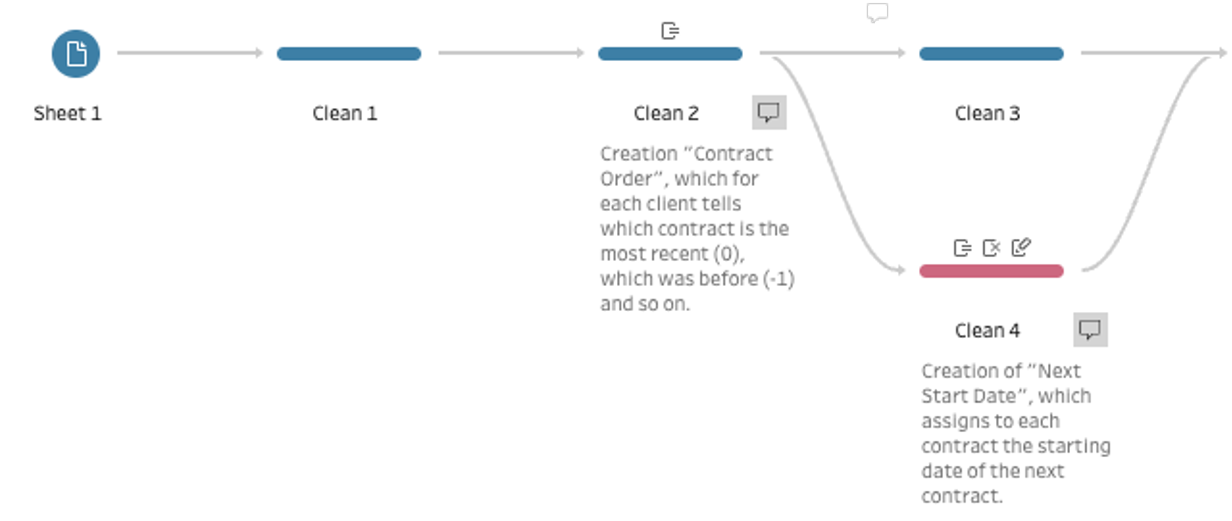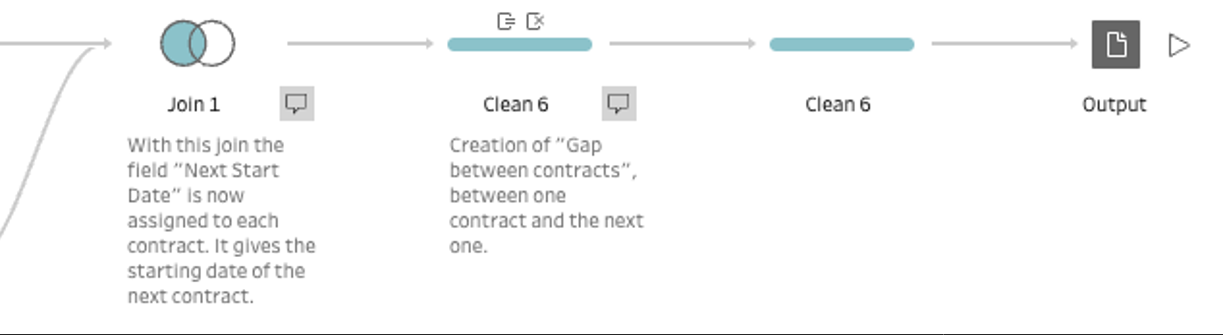The objective with Tableau Prep is to build a new field: the size of the gap between the end of one contract and the immediate next one. For that, we will need a field that shows the ordinal number of all contracts within a client (meaning: if it’s the first one, the second one, the third one, etc., also called ordinality) and a field that contains the starting date of the next contract. These steps might also be done on the database side to bring the size of the gap directly to Tableau.

Step 1: Create two calculated fields: “Contract Rank” & “Contract Order” (Clean 2)

Connect your data set and create a first calculated field named “Contract Rank” with the following logic:

`( { PARTITION [Client]: { ORDERBY [End Date]  DESC: RANK()}} )`

Then in a second field, use that rank field for this calculation, that we call “Contract Order”:

`([Contract Rank] - 1) * (-1)`

This first field will assign the ordinal number to the contracts of each client based on their end dates. Then, by subtracting 1 and multiplying by -1, the other field assigns a 0 to the current contract, a -1 to the previous contract, a -2 to the contract before that and so on.

Step 2: Split the flow and modify the previous calculated field (Clean 3 & 4)

Here we use a cool trick: we add another cleaning step, and put it below the other. With that our flow diverges  into two paths, which we’ll unify again later on. Add two clean steps from Clean 2. One will have no changes, and in the second, create another calculated field “Prev Contract”:

`[Contract Order] - 1`

This way we will be able to do a join of the table with itself, assigning each contract the start date of the immediate next one. Erase all fields except for [Prev Contract], [Client ID] and [Start Date], and rename that last one as “Next Start Date”.

Step 3: Build the Join

Build a join between the steps Clean 3 and Clean 4, using first [Client], then [Contract Order] and [Prev Contract] as keys. It must be a left join, so that the contracts with our calculated rank with 0 will not be filtered out. (There won’t be zeros there in our field [Prev Contract].)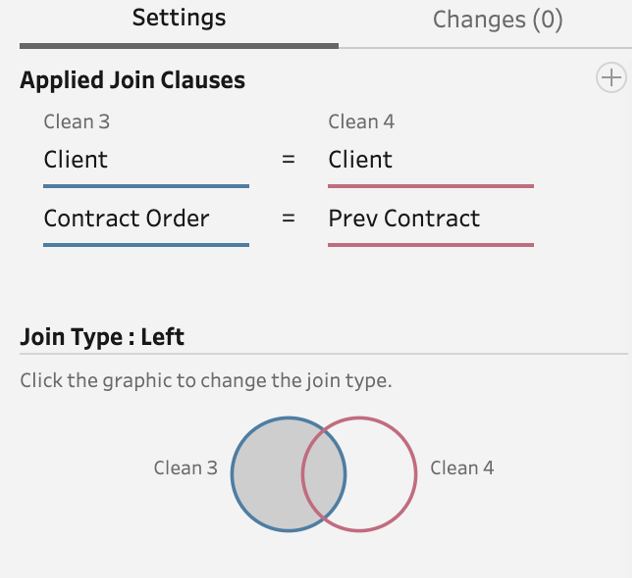Above: The necessary settings.

Step 4: Calculate the gap between contracts and create the extract

We will use the end date of each contract and the start date of the next contract to calculate this gap. For the case of the last contract (with our rank 0), it will be calculated against TODAY. That way, if even the last contract is too old (the gap is too wide), it might be discarded and no tenure calculation be performed at all.

```CASE [Contract Order]
WHEN 0 THEN DATEDIFF(‘day’, [End Date], TODAY())
ELSE DATEDIFF(‘day’, [End Date], [Next Start Date])
END```

The flow should look similar to this: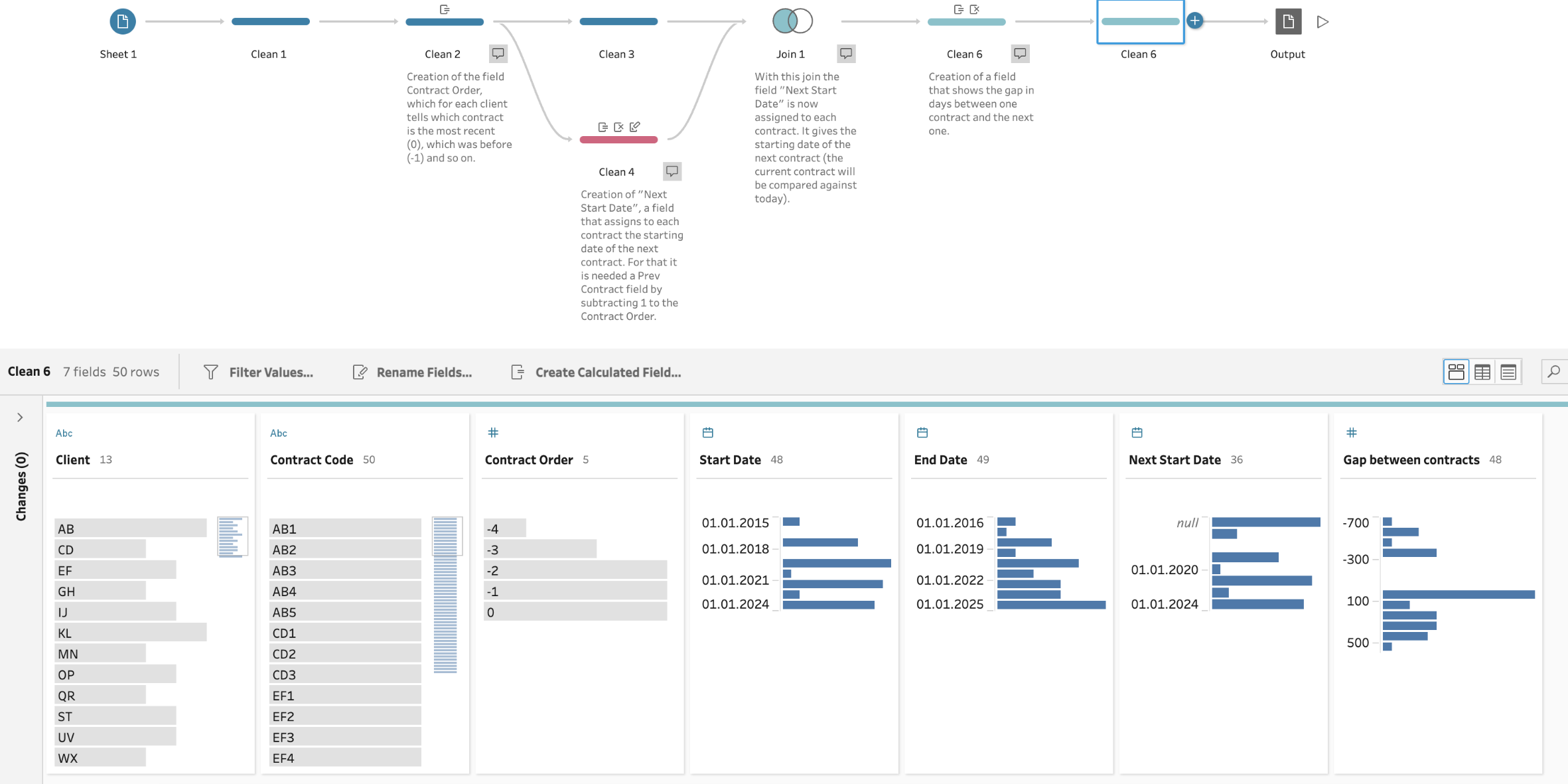Finally, create the output (here we go with an extract) and connect to it in Tableau Desktop.

### Part B: Calculated Fields and Parameter in Tableau Desktop

Now, that our data is set up in a usable way, we can start on the analytics side to solve our challenge. Everything down here is done in Tableau Desktop, but of course, this would also work in Tableau Web-Edit directly on the Tableau Server or on Tableau Cloud.

Step 5: Gap size parameter

Create a parameter that we are going to need for the next step. Use following settings: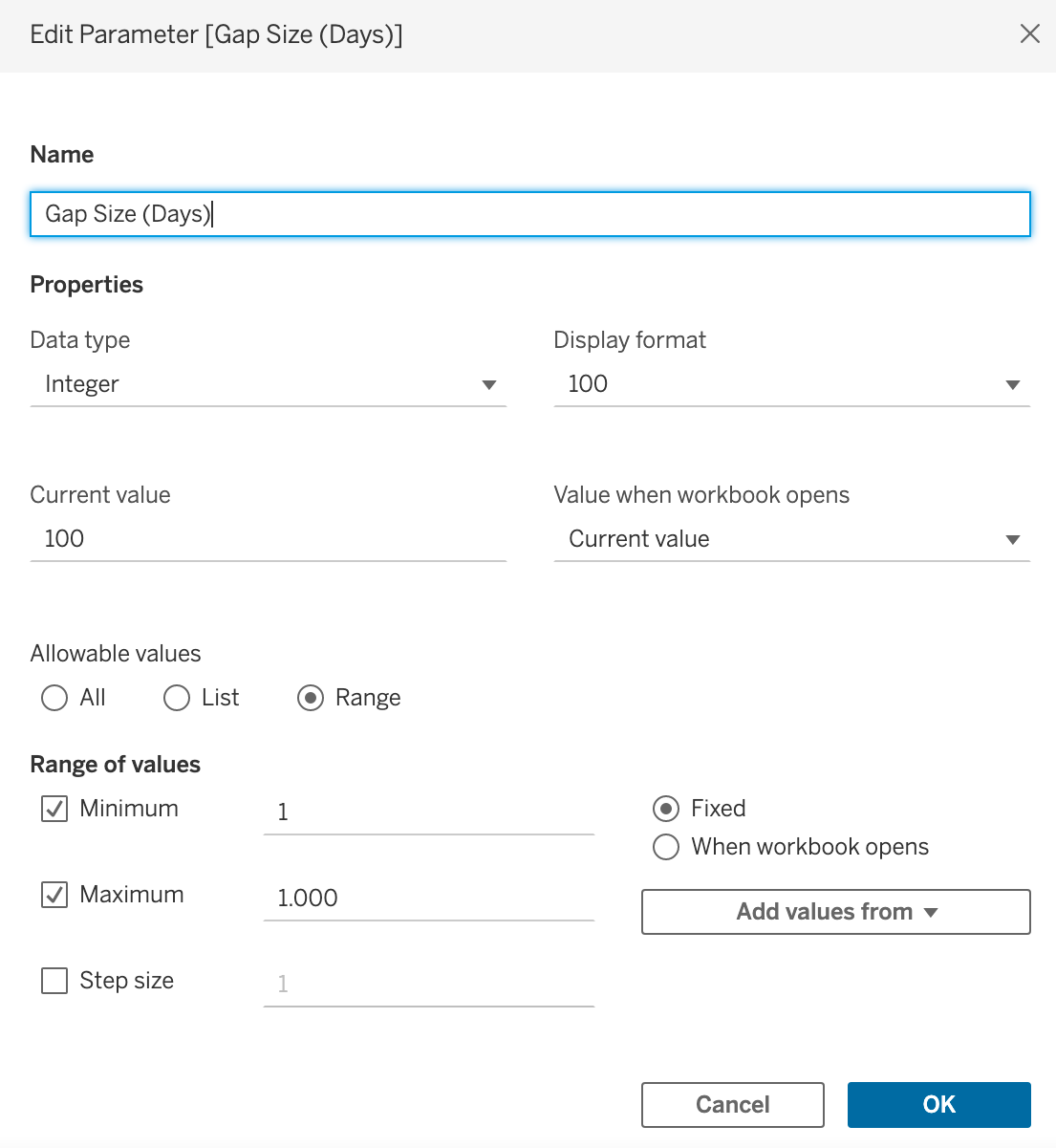Step 6: Create a calculated field “Contract connected”

This fields shows if the specified contract is connected to the next one depending on the size of the gap that we calculated before in Tableau Prep. Our parameter defines how big this gap may be. (In our example here: 100 days.):

```IF [Gap between contracts] <= [Gap Size (Days)]
THEN “Connected”
ELSE “Disconnected”
END```

Step 7: Create a calculated field “Connection lost contract”

This field will show the [Contract Order] if the contract is not connected. This is key, because this is the way we can identify the first contract in which the connection was lost, and up to that moment, the tenure should not be calculated any further:

```IF [Contract connected] = “Disconnected”
THEN [Contract Order]
END```

Step 8: Create a calculation “Number of contracts to include”

With this field, we can extract for which contract we first lost the connection to the next one. And then by multiplying its rank with -1, we get the number of contracts that should be taken into account. (The second part of the code.) If the connection was never lost, it means all contracts should be taken into account, so one has to simply count all contracts for that client (first part of the code):

```IF ISNULL( { FIXED [Client] : MAX([Connection lost contract]) } )
THEN { FIXED [Client] : COUNT([Contract Order]) }
ELSE ( { FIXED [Client] : MAX([Connection lost contract]) } ) * (-1)
END```

Up to this point, we would be able to build a table with the calculated fields to get a glimpse of what we’ve built: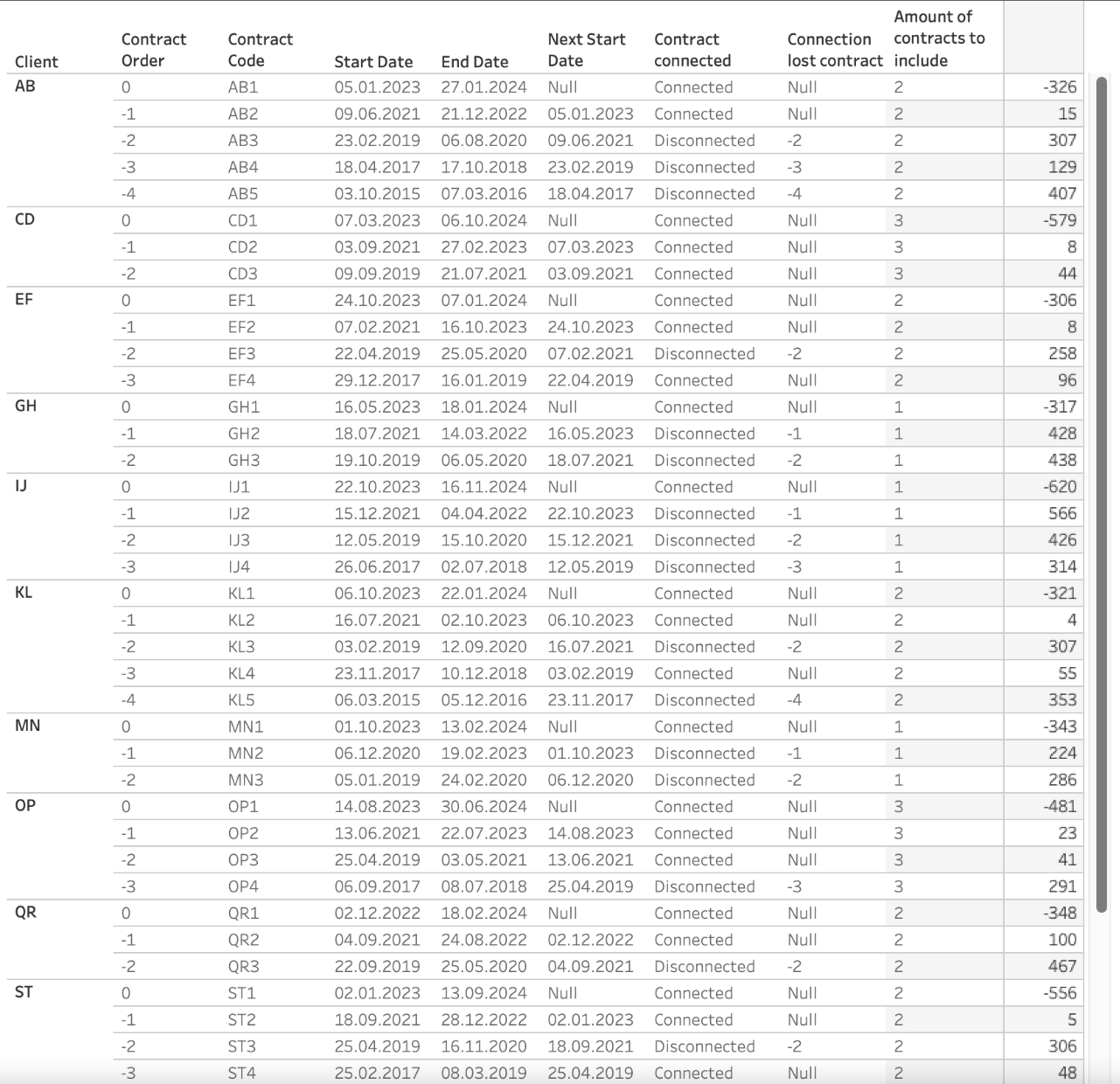Step 9: Create a calculation “Tenure Start Date”

For every client, we will get the starting date of its tenure by extracting the start date of the contract, whose order is the same as the number of contracts to include plus one, times minus one. E.g. if the number of contracts to include is three, then we would take the start date of the contract -2 (this would include contracts O, -1 and -2) and leave the rest outside:

```{ FIXED [Client] : MAX(
IF [Contract Order] = ([Amount of contracts to include] * (-1) + 1)
THEN [Start Date]
END
)}```

Step 10: Create a calculation “Tenure End Date”

The end tenure date is simply the end date of the last contract (or contract rank 0.) The second statement will exclude clients whose number of valid contracts is zero. That means that even the last contract is considered old and not valid anymore:

```{ FIXED [Client] : MAX(
IF [Contract Order] = 0 AND [Amount of contracts to include] > 0
THEN [End Date]
END
)}```

Step 11: Create a calculation “Tenure (months)”

LAST STEP! The tenure we are actually after, calculated by using the two respective dates we produced in the last steps:

`DATEDIFF(‘month’, [Tenure Start Date], [Tenure End Date])`

With that, we get our final table! It looks like this: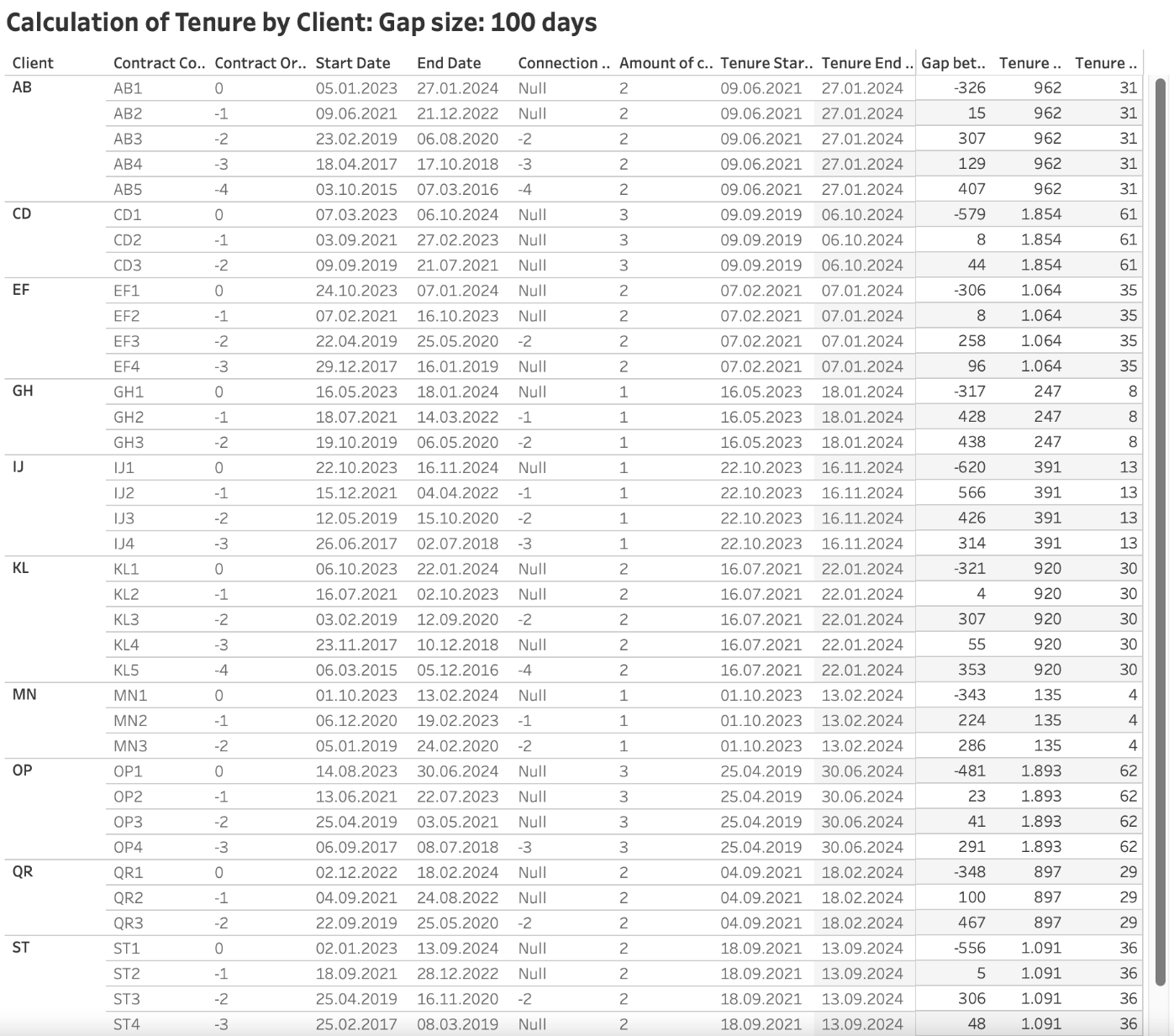And of course, we can put it into a sheet so it looks a bit more readable: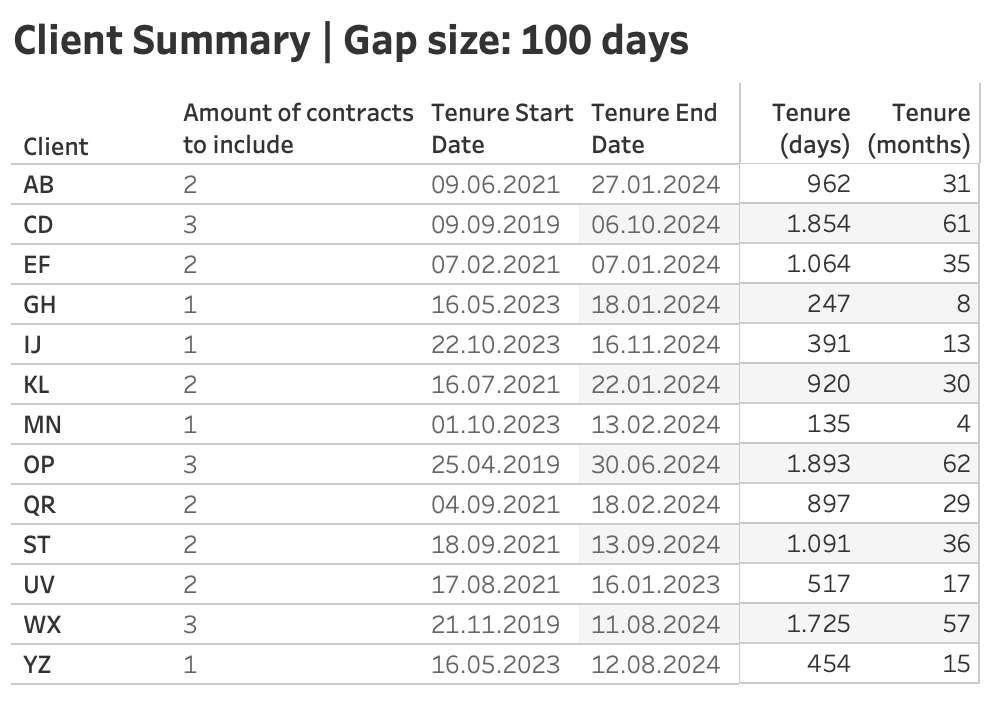Or even build a bar chart that visually shows when the tenure started and ends: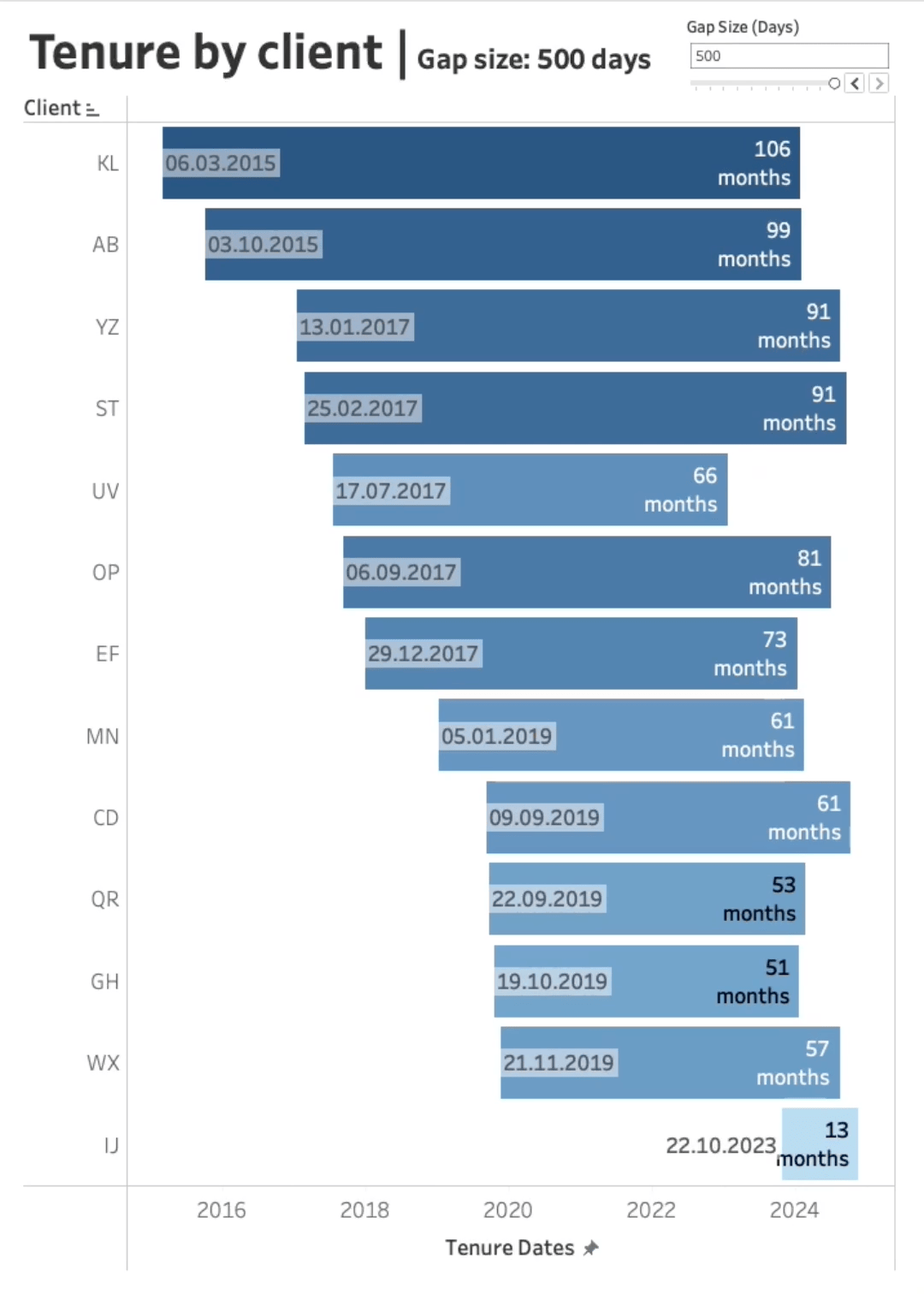That’s it! If you want to work with us on further Tableau projects, contact us.

### The Latest

• ##### Tableau Cloud System (SCIM): Unifying the Dimensions

Tenure Calculations in Tableau For those businesses that have a portfolio of clients, or a variety of members or students, a frequently asked question is, “For ...

See more from this author →

InterWorks uses cookies to allow us to better understand how the site is used. By continuing to use this site, you consent to this policy. Review Policy OK

×

Interworks GmbH
Ratinger Straße 9
40213 Düsseldorf
Germany
Geschäftsführer: Mel Stephenson

Kontaktaufnahme: markus@interworks.eu
Telefon: +49 (0)211 5408 5301

Amtsgericht Düsseldorf HRB 79752
UstldNr: DE 313 353 072